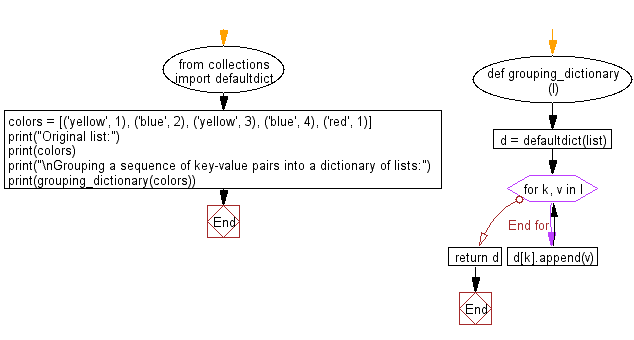﻿ Python: Grouping a sequence of key-value pairs into a dictionary of lists using collections module - w3resource# Python: Grouping a sequence of key-value pairs into a dictionary of lists using collections module

## Python Collections: Exercise-28 with Solution

Write a Python program to create a dictionary grouping a sequence of key-value pairs into a dictionary of lists. Use collections module.

Sample Solution:

Python Code:

``````from collections import defaultdict
def grouping_dictionary(l):
d = defaultdict(list)
for k, v in l:
d[k].append(v)
return d
colors = [('yellow', 1), ('blue', 2), ('yellow', 3), ('blue', 4), ('red', 1)]
print("Original list:")
print(colors)
print("\nGrouping a sequence of key-value pairs into a dictionary of lists:")
print(grouping_dictionary(colors))
```
```

Sample Output:

```Original list:
[('yellow', 1), ('blue', 2), ('yellow', 3), ('blue', 4), ('red', 1)]

Grouping a sequence of key-value pairs into a dictionary of lists:
defaultdict(<class 'list'>, {'yellow': [1, 3], 'blue': [2, 4], 'red': })
```

Flowchart:## Visualize Python code execution:

The following tool visualize what the computer is doing step-by-step as it executes the said program:

Python Code Editor:

Have another way to solve this solution? Contribute your code (and comments) through Disqus.

What is the difficulty level of this exercise?

Test your Programming skills with w3resource's quiz.

﻿

## Python: Tips of the Day

The Zip() Function:

```>>> students = ('John', 'Mary', 'Mike')
>>> ages = (15, 17, 16)
>>> scores = (90, 88, 82, 17, 14)
>>> for student, age, score in zip(students, ages, scores):
...     print(f'{student}, age: {age}, score: {score}')
...
John, age: 15, score: 90
Mary, age: 17, score: 88
Mike, age: 16, score: 82
>>> zipped = zip(students, ages, scores)
>>> a, b, c = zip(*zipped)
>>> print(b)
(15, 17, 16)
```